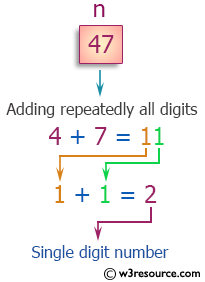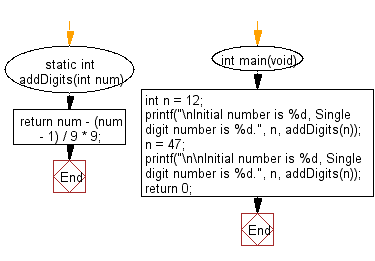﻿ C Program: Add digits until there is only one digit

# C Exercises: Add repeatedly all digits of a given non-negative number until the result has only one digit

## C Programming Mathematics: Exercise-12 with Solution

Write a C program to add repeatedly all digits of a given non-negative number until the result has only one digit.

Example:
Input: 48
Output: 2
Explanation: The formula is like: 4 + 8 = 12, 1 + 2 = 3.

Pictorial Presentation:Sample Solution:

C Code:

``````#include <stdio.h>

return num - (num - 1) / 9 * 9;
}

int main(void)
{
int n = 12;
printf("\nInitial number is %d, Single digit number is %d.", n, addDigits(n));
n = 47;
printf("\n\nInitial number is %d, Single digit number is %d.", n, addDigits(n));
return 0;
}
```
```

Sample Output:

```Initial number is 12, Single digit number is 3.

Initial number is 47, Single digit number is 2.
```

Flowchart:C Programming Code Editor:

Improve this sample solution and post your code through Disqus.

What is the difficulty level of this exercise?

Test your Programming skills with w3resource's quiz.

﻿